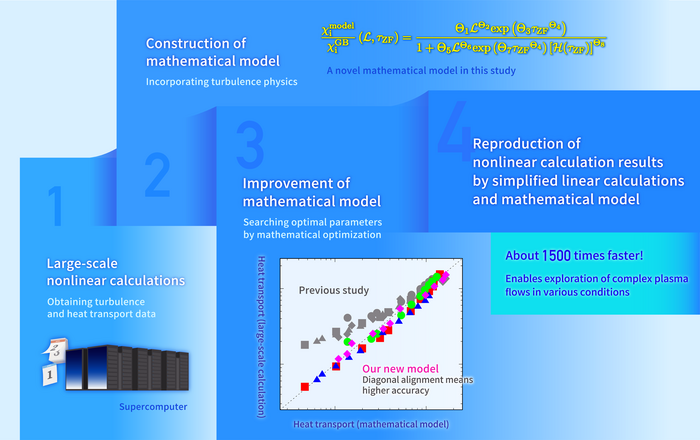News Release

# Simplified calculations reproduce complex plasma flows

Advances in theoretical studies on turbulence-driven heat transport in fusion plasms

Peer-Reviewed Publication

National Institutes of Natural Sciencesimage: Schematic diagram showing the research flow of constructing the mathematical model and the realization of high-speed computation by making full use of it. The graph in the figure indicates the high accuracy and wide applicability of the new mathematical model developed in this study. view more  Credit: National Institute for Fusion Science

Overview

Accurate and fast calculation of heat flow (heat transport) due to fluctuations and turbulence in plasmas is an important issue in elucidatingthe physical mechanisms and in predicting and controlling the performance of fusion reactors.

A research group led by Associate Professor Motoki Nakata of the National Institute for Fusion Science and Tomonari Nakayama, a Ph.D student at the Graduate University for Advanced Studies, has successfully developed a high-precision mathematical model to predict the heat transport level. This was achieved by applying a mathematical optimization method to a lot of turbulence and heat transport data obtained from large-scale numerical calculations using a supercomputer. This new mathematical model enables us to predict turbulence and heat transport in fusion plasmas only by simplified small-scale numerical calculations, which are approximately 1,500 times faster than conventional large-scale ones. This research result will not only accelerate research on fusion plasma turbulence, but also contribute to the study of various complex flow phenomena with fluctuations, turbulence and flows.

A paper summarizing this research result will be published in the online edition of Scientific Reports, an open access scientific journal, on March 16.

Research Background

In general, large-scale numerical calculations using supercomputers are indispensable to elucidate the physical mechanisms of complex structures and motions, such as atmospheric and ocean currents, neuronal signal transduction in the brain, and the molecular dynamics of proteins.

In a fusion reactor, high-temperature plasmas (high-temperature gaseous material in which electrons and nuclear ions are moving separately) are confined by magnetic fields, and a complex state called turbulence can occur in the plasma. The complex motion of vortices with various sizes cause heat flow (heat transport) in the turbulence. If the confined heat in the plasma is lost due to turbulence, the performance of the fusion reactor will be degraded, and thus plasma turbulence is one of the most important issues in fusion research.

Large-scale numerical calculations on supercomputers have been used to investigate the generation mechanism of the plasma turbulence, how to suppress it, and the heat transport due to the turbulence. Nonlinear calculations are used to solve the equations of motion of the plasma. However, since turbulence varies depending on the plasma state, an enormous amount of computational resources is required to carry out large-scale nonlinear calculations for the entire plasma region with a variety of states. There has been much research attempting to reproduce the results of nonlinear calculations by simplified theoretical models or small-scale numerical calculations, but the degraded accuracy for different plasma conditions and the limited range of applications still remain to be improved. Therefore, a new mathematical model that can solve these issues us expected to be realized.

Research Results

A research group led by Associate Professor Motoki Nakata of the National Institute for Fusion Science and Tomonari Nakayama, a Ph.D student of the Graduate University for Advanced Studies, Professor Mitsuru Honda of Kyoto University, Dr. Emi Narita of the National Institute for Quantum Science and Technology, Associate Professor Masanori Nunami and Assistant Professor Seikichi Matsuoka of the National Institute for Fusion Science has conducted a study on a novel method to reproduce the nonlinear calculation results of turbulence and heat transport by “linear” calculations, which are small-scale ones based on a simplified equation of motion. Thus, a high-speed and high-accuracy prediction with wider applicability has been achieved.

First, Prof. Nakata and his colleagues performed a number of large-scale nonlinear calculations to analyze turbulence at multiple locations in the plasma and at many temperature distribution states, and obtained the data on the turbulence intensity and heat transport level. They then proposed a simplified mathematical model based on physical considerations to reproduce it. This contained eight tuning parameters, and it was necessary to find their optimal values to best reproduce the data from the large-scale nonlinear calculations. Mr. Nakayama, a graduate student, searched for the optimal values among a huge number of combinations by applying mathematical optimization techniques used in path finding and machine learning, etc. As a result, he succeeded in constructing a new mathematical model that maintains high accuracy and greatly expands the range of applicability compared to that  used in previous research.

By combining this mathematical model with linear calculations for plasma instabilities, it is now possible to predict plasma turbulence and heat transport level with high accuracy, about 1,500 times faster than conventional large-scale nonlinear calculations (Figure).

Significance of the Results and Future Developments

The newly constructed fast and accurate mathematical model will greatly accelerate research on turbulence in fusion plasmas. In addition, the model will also advance research on integrated simulations, combining the mathematical model of turbulence and numerical simulations of the other phenomena (e.g., temporal variations in temperature and density distribution, confinement magnetic field, etc.) in order to analyze the entire fusion plasma field. In addition, the model is expected to contribute to the elucidation of the mechanism of suppressing turbulence-driven heat transport, and to make a significant contribution to research towards innovative fusion reactors based on such a mechanism.

The challenge of predicting "complexity" from "simplicity" is a common issue in various sciences and technologies that deal with complex structures and dynamics. In the future, we will apply the modeling methods developed in this research to the study of complex flows, not limited to fusion plasmas.

Disclaimer: AAAS and EurekAlert! are not responsible for the accuracy of news releases posted to EurekAlert! by contributing institutions or for the use of any information through the EurekAlert system.